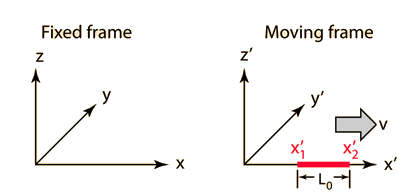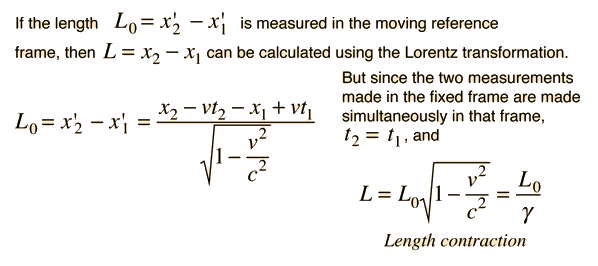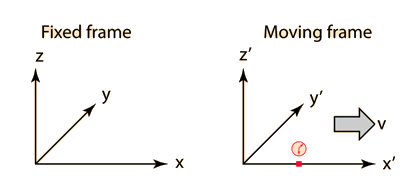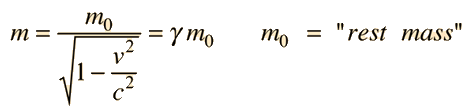# Length ContractionThe length of any object in a moving frame will appear foreshortened in the direction of motion, or contracted. The amount of contraction can be calculated from the Lorentz transformation. The length is maximum in the frame in which the object is at rest.For v = c, L = L0
 Lorentz transformation
 Application in muon decay experiment
Index

Relativity concepts

 HyperPhysics***** Relativity R Nave
Go Back

# Time DilationA clock in a moving frame will be seen to be running slow, or "dilated" according to the Lorentz transformation. The time will always be shortest as measured in its rest frame. The time measured in the frame in which the clock is at rest is called the "proper time".For v = c, T = T0

For small velocities at which the relativity factor is very close to 1, then the time dilation can be expanded in a binomial expansion to get the approximate expression:Time dilation experiments
 A brief overview of time.
Index

Relativity concepts

 HyperPhysics***** Relativity R Nave
Go Back

# "Relativistic Mass"

The increase in "effective mass" with speed is given by the expressionIt follows from the Lorentz transformation when collisions are described from a fixed and moving reference frame, where it arises as a result of conservation of momentum. You can also approach it from the relativistic energy expressionwhere the effective mass is given by the energy divided by c2. This suggests that this "m" is really about the energy, and that the real mass is the rest mass m0.

For v = c, m =m0

The increase in relativistic "effective mass" is associated with speed of light c the speed limit of the universe. This increased effective mass is evident in cyclotrons and other accelerators where the speed approaches c. Exploring the calculation above will show that you have to reach 14% of the speed of light, or about 42 million m/s before you change the effective mass by 1%.

This language about "effective mass" is included here because it has long been used in elementary textbooks to describe the increase in energy with velocity, and it has a certain appeal for the description of relativistic effects such as the Cambridge example. But you won't find it in modern physics textbooks. One comment from a classic work on relativity is

"Ouch! The concept of 'relativistic mass' is subject to misunderstanding. That's why we don't use it. First, it applies the name mass - belonging to the magnitude of a 4- vector - to a very different concept, the time component of a 4-vector. Second, it makes increase of energy of an object with velocity or momentum appear to be connected with some change in internal structure of the object. In reality, the increase of energy with velocity originates not in the object but in the geometric properties of space-time itself." (Taylor and Wheeler in Spacetime Physics)

 Cambridge example Problems with variable mass concept Mass of photon?
Index

Relativity concepts

 HyperPhysics***** Relativity R Nave
Go Back

# "Relativistic Mass" Example

At the electron accelerator in Cambridge, Mass., the final acceleration stage has the following characteristics:

 Feed electrons Electrons out Velocity 0.99986 c 0.999999996 c "Mass" 60 m0 11,180 m0 Relative time for auto trip 2 hr 1 hr 59 min 59 sec

This increase in velocity requires a 186x increase in energy, yet only saves one second off a two hour journey.

### Problems with variable mass concept

Index

Relativity concepts

Reference Zukav

 HyperPhysics***** Relativity R Nave
Go Back

# Problems with variable mass

Even though circumstances like that described at the Cambridge accelerator are conveniently described by assuming an increasing mass, that is not the only way to describe these experiments, and there are problems with the concept of variable relativistic mass. Einstein's point of view is described in the following quote:

 "It is not good to introduce the concept of the mass of a moving body for which no clear definition can be given. It is better to introduce no other mass concept than the 'rest mass' m. Instead of introducing M it is better to mention the expression for the momentum and energy of a body in motion."Upon being introduced to special relativity for the first time, it is easier to contemplate concepts like the speed of light as the speed limit of the universe by envisioning the mass as increasing to infinity at velocity c. However, when one has become familiar with the concepts of relativistic momentum and relativistic energy, there is no real need for the variable mass concept.

Index

Relativity concepts

 HyperPhysics***** Relativity R Nave
Go Back